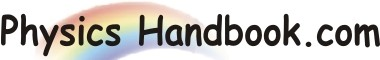HOME TOPICS DEFINITIONS TABLES LAWS INVENTIONS EXPERIMENTS QUIZ VIDEOS
 A B C D E F G H I J K L M N O P Q R S T U V W X Y Z
Relative Permittivity
Permittivity is the property of a medium and affects the magnitude of force between two point charges.
Air or vacuum has minimum value of permittivity. The absolute (or actual) permittivity of air or vacuum is 8.854 x 10-12 C2 N-1 m-2.
The absolute permittivity ε of all other insulating materials is greater than ε0. The ratio ε / ε0 is called relative permittivity of the material and is denoted by K (or εr).
K = ε / ε0  =  Absolute permittivity of medium / Absolute permittivity of air
It may be noted that the relative permittivity is also called dielectric constant.
Force between two charges in air is,
Fair  =  (1 / 4 x π x ε0 ) x (q1 x q2 / r2)
Force between the same two charges held same distance apart in a medium of absolute permittivity ε is
Fm  =  (1 / 4 x π x ε ) x (q1 x q2 / r2)
=  (1 / 4 x π x ε0 x K ) x (q1 x q2 / r2)
Fair / Fm  =  ε / ε0  =  K  =  Relative permittivity of the medium
Hence, relative permittivity of a medium may be defined as the ratio of force between two charges separated by a certain distance in air to the force between the same charges separated by the same distance in the medium.
For air or vacuum, K  =  ε / ε0  =  1. For all other insulating materials, the value of k is more than 1.
Fm  =  Fair / K. This implies that force between two charges is decreased when air is replaced by other insulating medium.
K is a number, being the ratio of two absolute permittivities.